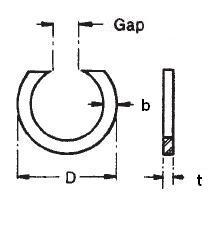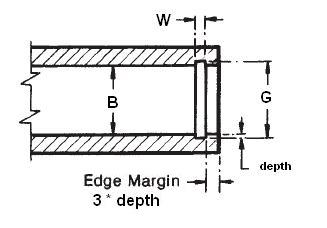### NAN-600

Display Units: inches |  metric
Request Quick Quote for this part.
 Ring Specs (D) Free Diameter: 6.309 +0.125 / -0.000 in. (t) Thickness: 0.125 +0.002 / -0.002 in. (b) Radial Wall: 0.312 +0.005 / -0.005 in. Groove Specs: (B) Application Diameter: 6.000 in. (G) Groove Diameter: 6.204 +0.007 / -0.007 in. (W) Groove Width: 0.139 +0.006 / -0.000 in. Groove Depth: 0.102 in. Other Specs Approximate Weight per 1000: (Gap Max) Maximum Free Gap: 1.500 in. (Gap Min) Minimum Free Gap: 1.000 in. (Pg) Theoretical Thrust Load Capacity - Groove Yield:Notes: Yield Strength of Groove Material (Ys): 45,000 psi. Calculated using a safety factor (K) of 4Equation:Pg = [ B * d * Ys * pi ] / K    = [ (6.000 in.) * (0.102 in.) * (45,000 psi) * 3.14 ] / 4 21,618 lbs. (Pr) Theoretical Thrust Load Capacity - Ring Shear:Notes: Ring Material: Carbon Spring Steel (SAE 1060-1095). Shear Strength of Ring Material (Ss): 153,000 psi. Calculated using a safety factor (K) of 3Equation:Pr = [ B * t * Ss * pi ] / K    = [ (6.000 in.) * (0.125 in.) * (153,000 psi) * 3.14 ] / 3 120,105 lbs. Industry Equivalent Part Number(s):NAN-600